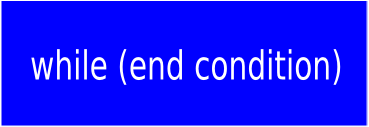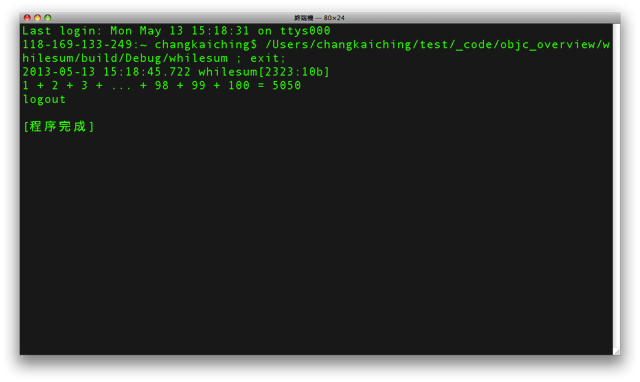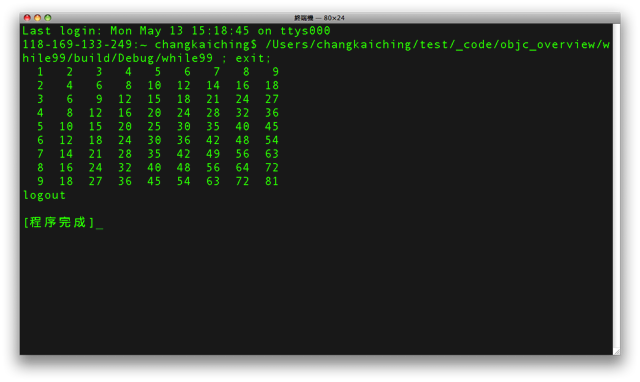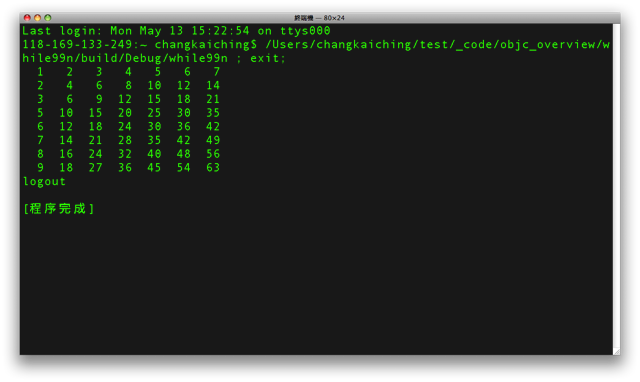## 公告

《如何自學程式設計》入選 Google Play 台灣地區2016年度最佳書籍｜本站於 Google Play 銷售電子書。

------
Udemy ｜ Python 專案開發入門的二十一堂課 | 促銷優惠

### Objective-C 快速導覽 - while 迴圈結構

 1. 控制變數初始設定 2. 迴圈結束條件測試 3. 調整控制變數的值```#import <Foundation/Foundation.h>

int main (int argc, const char * argv[]) {
NSAutoreleasePool * pool = [[NSAutoreleasePool alloc] init];

int sum = 0;
int i = 1;

while (i <= 100) {
sum += i++;
}

NSLog(@"\n1 + 2 + 3 + ... + 98 + 99 + 100 = %d", sum);

[pool drain];
return 0;
}

/* 《程式語言教學誌》的範例程式
http://pydoing.blogspot.com/
檔名：whilesum.m
功能：Objective-c 程式範例
作者：張凱慶
時間：西元 2013 年 4 月 */``````#import <Foundation/Foundation.h>

int main (int argc, const char * argv[]) {
NSAutoreleasePool * pool = [[NSAutoreleasePool alloc] init];

int i = 1;
int j = 1;

while (i <= 9) {
while (j <= 9) {
printf("%3d ", i * j);
j++;
}

j = 1;
i++;
printf("\n");
}

[pool drain];
return 0;
}

/* 《程式語言教學誌》的範例程式
http://pydoing.blogspot.com/
檔名：while99.m
功能：Objective-c 程式範例
作者：張凱慶
時間：西元 2013 年 4 月 */``````#import <Foundation/Foundation.h>

int main (int argc, const char * argv[]) {
NSAutoreleasePool * pool = [[NSAutoreleasePool alloc] init];

int i = 1;
int j = 1;

while (i <= 9) {
if (i == 4) {
i++;
continue;
}

while (j <= 9) {
if (j == 8) {
break;
}

printf("%3d ", i * j);
j++;
}

j = 1;
i++;
printf("\n");
}

[pool drain];
return 0;
}

/* 《程式語言教學誌》的範例程式
http://pydoing.blogspot.com/
檔名：while99n.m
功能：Objective-c 程式範例
作者：張凱慶
時間：西元 2013 年 4 月 */```while 迴圈也可利用 for 迴圈來取代，反之亦然。

Objective-C 快速導覽
Objective-C 教材

Programming with Objective-C: About Objective-C
Programming with Objective-C: Working with Objects